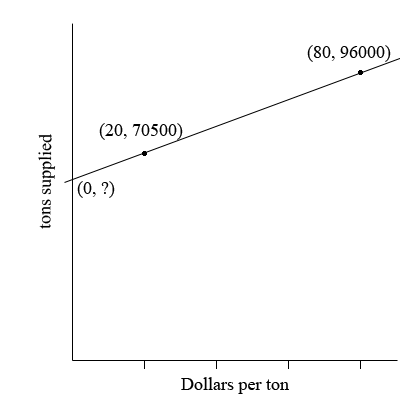SEARCH HOMEMath Central Quandaries & QueriesQuestion from Jourdan: Suppose a mining company will supply 96000 tons of ore per month if the price is 80 dollars per ton but will supply 70500 tons per month if the price is 20 dollars per ton. Assuming the supply function is of the form y = m x + b , find the slope, m and y-intercept, bHi Jourdan,

I drew a diagram containing the two points from your question.The third point, the one with X-coordinate $0$ has an unknown Y-coordinate, the Y-intercept. Use the method in my response to Conor to find the slope of the line, $m,$ and then Expression 2 in the same page to find $b,$ the Y-intercept.

PennyMath Central is supported by the University of Regina and The Pacific Institute for the Mathematical Sciences.